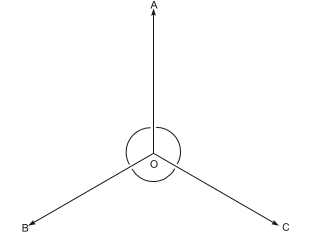# Value-Based Questions: Lines & Angles Notes | Study Mathematics (Maths) Class 9 - Class 9

## Class 9: Value-Based Questions: Lines & Angles Notes | Study Mathematics (Maths) Class 9 - Class 9

The document Value-Based Questions: Lines & Angles Notes | Study Mathematics (Maths) Class 9 - Class 9 is a part of the Class 9 Course Mathematics (Maths) Class 9.
All you need of Class 9 at this link: Class 9

Question 1. On Monday Prashant’s school bus was late due to a traffic jam and his maths class was missed. He was very upset as his teacher had introduced a new topic on geometry. Rahul promised to help him after school. Rahul went to Prashant’s house and explained the topic. He also gave him the following test also: Which of the following statements are true?

(a) Two adjacent angles are said to form a linear pair of angles if their uncommon arms are two opposite rays.

(b) The sum of all the angles around a point is equal to 180°.

(c) The measure of an angle is twice the measure of its supplementary angle. Its measure is 60°.

(d) The angle between the bisectors of a linear pair of angles is a right angle.

(e) Three or more lines are said to be concurrent if there is a point that lies on all of them.
(i) Write the appropriate answers to the above questions.
(ii) Which mathematical concept is used in the above problem?
(iii) By explaining the topic to Prashant, which value is depicted by Rahul?
Solution.
(a) True
(b) False
(c) False
(d) True
(e) True
(ii) Lines and Angles (Geometry).
(iii) Helping a friend in need.

Question 2. Three friends plan to help flood victims. They move away from a point in three different directions such that the direction of each is equally inclined to those of the other two:
(i) Find the angles their directions make with another.
(ii) Which mathematical concept is used in the above problem?
(iii) By helping the flood victims, which value is depicted by the teachers and students?
Solution.
(i) Let ‘O’ be the point from where the three friends move to the points A, B and C, such that OA makes equal angles with OB and OC such that
∠AOB = ∠AOC                       ..... (i)
Also, OB makes equal angles with OA and OCSuch that
∠AOB = ∠BOC                   ..... (ii)
From (i) and (ii), we get
∠AOB = ∠BOC = ∠AOC             ..... (iii)
Since the sum of all angles around a point = 360°
⇒ ∠AOB + ∠BOC + ∠AOC = 360°
⇒ ∠AOB + ∠AOB + ∠AOB = 360°               [from iii]
⇒ 3 ∠AOB = 360°
⇒ ∠AOB = (360°/3)= 120°
∴ From (iii), we have: ∠BOC = 120° and ∠AOC = 120°

(ii) Lines and Angles (Geometry)

(iii) Helping attitude towards disastrous victims.

The document Value-Based Questions: Lines & Angles Notes | Study Mathematics (Maths) Class 9 - Class 9 is a part of the Class 9 Course Mathematics (Maths) Class 9.
All you need of Class 9 at this link: Class 9Use Code STAYHOME200 and get INR 200 additional OFF

## Mathematics (Maths) Class 9

73 videos|351 docs|110 tests

Track your progress, build streaks, highlight & save important lessons and more!

,

,

,

,

,

,

,

,

,

,

,

,

,

,

,

,

,

,

,

,

,

;